# ISEE Lower Level Math : How to find the points on a coordinate plane

## Example Questions

### Example Question #1 : Coordinate Geometry

In which quadrant is the point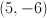located in?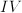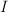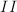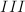Explanation:

From the originis to the right 5 and down 6 making it in quadrant IV.

### Example Question #11 : Coordinate Geometry

Angela plotted points A through E on the coordinate plane shown below.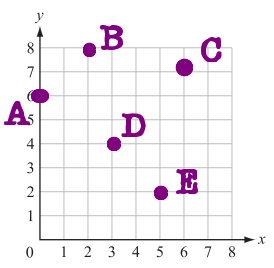What are the coordinates for point B?

(7, 2)

(2, 7)

(8, 8)

(2, 8)

(8, 2)

(2, 8)

Explanation:

Begin at point (0, 0) in the bottom left hand corner of the coordinate plane. From there, count the number of spaces you move to the right to line up with Point B. This will be the first number in the ordered pair. Next, count the number of spaces to move up until you reach Point B. This will be the second number in your ordered pair.

You should have counted 2 spaces to the right and 8 spaces up. The ordered pair for Point B is (2, 8).

### Example Question #11 : Geometry

Angela plotted points A through E on the coordinate plane shown below.What are the coordinates for Point A?

(0, 5)

(1, 6)

(6, 0)

(6, 1)

(0, 6)

(0, 6)

Explanation:

Begin at point (0, 0) in the bottom left hand corner of the coordinate plane. From there, count the number of spaces you move to the right to line up with Point A. This will be the first number in the ordered pair. Next, count the number of spaces to move up until you reach Point A. This will be the second number in your ordered pair.

You should have counted 0 spaces to the right and 6 spaces up. The ordered pair for Point B is (0, 6).

### Example Question #942 : Isee Lower Level (Grades 5 6) Mathematics Achievement

Angela plotted points A through E on the coordinate plane shown below.Which point is located at the ordered pair (6, 7)?

Point C

Point D

Point A

Point E

Point B

Point C

Explanation:

Start at point (0, 0) in the bottom, left corner of the the coordinate plane. From there, count right 6 spaces. This is the first number in the given ordered pair. From that point, count up 7 spaces. This is the second number in the given ordered pair.

By moving right 6 and up 7, you should have found Point C.

### Example Question #2 : How To Find The Points On A Coordinate Plane

Angela plotted points A through E on the coordinate plane shown below.Which point is located at the ordered pair (2, 8)?

Point C

Point A

Point D

Point B

Point E